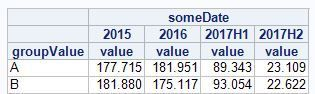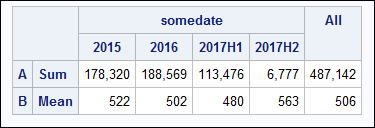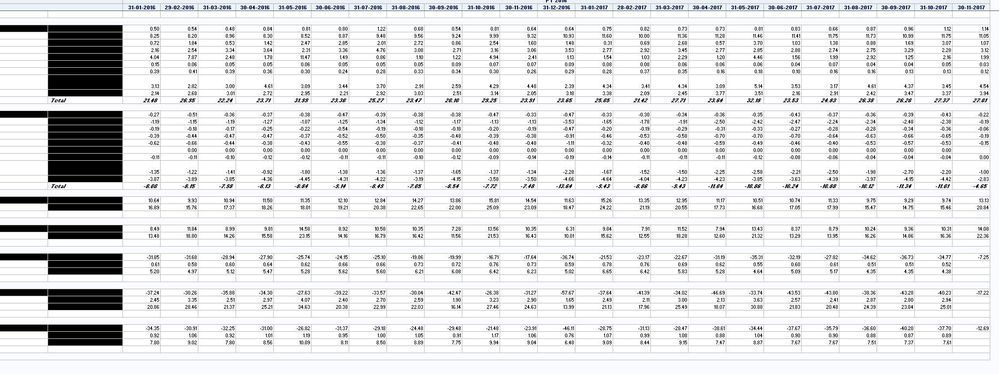## PROC REPORT - Calculation of Mean and SUM based on row

Dear all,

I have this sample data giving me this sample report.  I have row A and row B.  I would like the calculations to be either Mean or Sum based on the Row values.  ie if in the first column row is A then Sum if it is B then mean.

``````data have;
do groupValue = "A", "B";
do someDate = intnx("year", today(), -2, "B") to mdy(8,15, year(today()));
value = ceil(ranuni(0) * 1000);
output;
end;
end;
format
someDate date9.
value commax14.
;
run;

proc format;
value year_current
low - "31dec2016"d = [year4.]
"01jan2017"d - "31jul2017"d = "2017H1"
"01aug2017"d - "31dec2017"d = "2017H2"
;
run;

proc report data=have;
column groupValue someDate, value;
define groupValue / group;
define someDate / across format=year_current.;
run;``````

Which gives me:I would like the values in row A to be means and have the row B as sums (as it already is).

Regards,

1 ACCEPTED SOLUTION

Accepted Solutions

## Re: PROC REPORT - Calculation of Mean and SUM based on row

This would be very simple to do if you use data steps plus PROC MEANS/PROC SUMMARY to obtain the desired results before you get to PROC REPORT. Then you could use PROC REPORT to simply produce the report, and you are not using PROC REPORT to do the calculations.

--
Paige Miller
5 REPLIES 5

## Re: PROC REPORT - Calculation of Mean and SUM based on row

This would be very simple to do if you use data steps plus PROC MEANS/PROC SUMMARY to obtain the desired results before you get to PROC REPORT. Then you could use PROC REPORT to simply produce the report, and you are not using PROC REPORT to do the calculations.

--
Paige Miller

## Re: PROC REPORT - Calculation of Mean and SUM based on row

Hi:

Or, as an alternate approach, another idea is to switch to PROC TABULATE. If you run this example, which uses SASHELP.SHOES, you'll see that a separate statistic was calculated for every row:

``````proc tabulate data=sashelp.shoes f=comma18.;
var sales inventory returns;
class region;
table sales*sum inventory*mean returns*max,
region all;
run;``````

You might have to restructure your data a bit to get this, but it is very do-able. So, for example, if your existing structure of data were in a file called have.csv (attached), then you could product this output:With this code:

``````data have ;
infile 'c:\temp\have.csv' dlm=',' dsd;
input groupvalue \$ somedate : date9. value;
format someDate date9. value commax14. ;
run;

proc sort data=have; by somedate groupvalue;
run;

proc transpose data=have out=hav_out(drop=_name_);
by somedate;
id groupvalue;
var value;
run;

proc tabulate data=hav_out f=comma18.;
var a b;
class somedate;
table a*sum b*mean,
somedate all;
format somedate year_current.;
run;``````

## Re: PROC REPORT - Calculation of Mean and SUM based on row

Thank you very much to both, I will try both approaches.  I am a bit hesitant to use PROC Tabulate as my real code groups the rows and adds aggregates (totals) after certain groups.  I would like to keep this.  Can Proc Tabulate replicate this as well.  Not very versed in this Procedure.

``````PROC REPORT DATA=WORK.RANK_ALL;
COLUMNS Rank Grouping REGROUPING Amounts, Summary_date ('Overall' AMOUNTS = TotalAmount) _dummy ;
WHERE SEGMENT_Lvl_1 EQ 'Bespoke' AND YEAR(SUMMARY_DATE)ne 2017;
DEFINE Rank / Group '' NOPRINT;
DEFINE Grouping / GROUP ORDER=INTERNAL '' LEFT;
DEFINE REGROUPING / GROUP '' ;
DEFINE SUMMARY_DATE / ACROSS ORDER=INTERNAL '' FORMAT=DDMMYYd10.; /*mmyyd.*/
DEFINE AMOUNTS / ANALYSIS SUM '' FORMAT=COMMA20.2;
DEFINE _dummy / COMPUTED '' NOPRINT;
DEFINE TotalAmount / SUM 'Total' NOPRINT;
BREAK AFTER Rank / SUMMARIZE STYLE={foreground=black fontweight=bold fontstyle=italic};
COMPUTE _dummy / CHAR LENGTH = 21;
_dummy = catx(":", Rank, _break_);
IF lowcase(_break_) = "rank" AND Rank NOT IN ('a','b','e','f','i','j','k','l','m') THEN DO;
REGROUPING = "Total";
CALL MISSING(Rank);
END;
IF lowcase(_break_) = "rank" AND Rank IN ('a','b','e','f','i','j','k','l','m') THEN DO;
CALL MISSING(Rank, REGROUPING);
CALL MISSING(_c3_,_c4_,_c5_,_c6_,_c7_,_c8_,_c9_,_c10_,_c11_,_c12_,_c13_,_c14_,_c15_,_c16_,_c17_,_c18_,_c19_,_c20_,_c21_,_c22_,_c23_,_c24_,_c25_,_c26_);
END;

ENDCOMP;
COMPUTE AFTER Rank;
LINE '';
ENDCOMP;

RUN;``````

Which gives me this output:As you can see I am adding totals to certain groups and not others.  I will have a look at PROC Tabulate and see if I can create this output.  Perhaps I should just work on my source data and get that aligned first to make it easier. My goal was to group the dates as Years and Quarters which I have now achieved thanks to a community member.  The only thing left is to sum or mean based on the grouping.  I will give PROC Tabulate a try.

Thanks all again,

Aksel

## Re: PROC REPORT - Calculation of Mean and SUM based on row

Hi:
TABULATE can't do what you're doing with PROC REPORT.
cynthia

## Re: PROC REPORT - Calculation of Mean and SUM based on row

Thank you very much as always.

Discussion stats
• 5 replies
• 2100 views
• 2 likes
• 3 in conversation